# Question & Answer: In java, using the pseudocode as references provided below create a functions that will create the bodie…..

In java, using the pseudocode as references provided below create a functions that will create the bodies of an undirected graph. The pseudocode will help.

Functions

Don't use plagiarized sources. Get Your Custom Essay on
Question & Answer: In java, using the pseudocode as references provided below create a functions that will create the bodie…..
GET AN ESSAY WRITTEN FOR YOU FROM AS LOW AS \$13/PAGE

1. dpt() – provides the output of the forest from depth first tree.

2. bft – provides the output of the forest from bread first traversal

3. articulationpoint() – returns all the values of the articulation point from the set in A

4. Bridges() – obtains and therefore provides the value of all bridges in A

5. distances(a) – obtains the output by computing the distance between each vertices from a

6. connects() – returns the output of the forests by providing sets of strongly connected units of A

Let A = (V, E ) be a graph (either directed or undirected).

Initialize FIFO queue Q as being empty.

Initialize each vertex of G as being unmarked.

While there exist unmarked vertices:

Let u be one such unmarked vertex.

Mark u and place it in Q.

While Q is nonempty:

Remove node u from Q.

For every v that is a neighbor/child of u:

If v is unmarked, then mark v and place it in Q

Depth-First Graph Traversal Algorithm.

Let A= (V, E ) be a graph (either directed or undi-

rected).

Initialize stack S as being empty.

Initialize each vertex of A as being unmarked.

While there exist unmarked vertices:

Let u be one such unmarked vertex.

Mark u and push it on to S.

While S is nonempty:

Let u be at the front of S.

Let v be the first unmarked neighbor/child of u.

If v does not exist:

Pop u from S.

Otherwise:

Mark v and push v on to S.

ijkstra’s algorithm for a distance traversal of a Graph from source vertex

s

.

Let G = ( V, E, c ) be a network with nonnegative edges, and s ∈ V a vertex from which the algorithm begins.

Add the elements of V to an initially empty min Heap H.

Give s priority p(s) = 0, and all other vertices infinite priority.

Set parent(s) = NULL.

While H is nonempty:

Pop node u from H.

Set d(s,u) = p(u).

If p(u) < INFINITY:

For every v that is a child of u:

If d(s,u) + c(u,v) < p(v):

Set p(v) = p(u) + c(u,v), and adjust H.

Set parent(v) = u.

The depth and breadth first traversals are listed individully..

articulationpoint()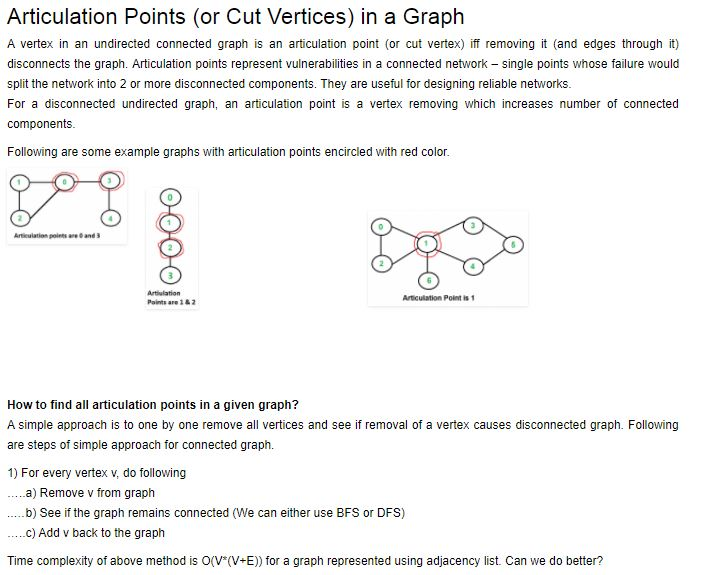code:

// A Java program to find articulation points in an undirected graph
import java.io.*;
import java.util.*;

// This class represents an undirected graph using adjacency list
// representation
class Graph
{
private int V; // No. of vertices

// Array of lists for Adjacency List Representation
int time = 0;
static final int NIL = -1;

// Constructor
Graph(int v)
{
V = v;
for (int i=0; i<v; ++i)
}

//Function to add an edge into the graph
{
}

// A recursive function that find articulation points using DFS
// u –> The vertex to be visited next
// visited[] –> keeps tract of visited vertices
// disc[] –> Stores discovery times of visited vertices
// parent[] –> Stores parent vertices in DFS tree
// ap[] –> Store articulation points
void APUtil(int u, boolean visited[], int disc[],
int low[], int parent[], boolean ap[])
{

// Count of children in DFS Tree
int children = 0;

// Mark the current node as visited
visited[u] = true;

// Initialize discovery time and low value
disc[u] = low[u] = ++time;

// Go through all vertices aadjacent to this
while (i.hasNext())
{
int v = i.next(); // v is current adjacent of u

// If v is not visited yet, then make it a child of u
// in DFS tree and recur for it
if (!visited[v])
{
children++;
parent[v] = u;
APUtil(v, visited, disc, low, parent, ap);

// Check if the subtree rooted with v has a connection to
// one of the ancestors of u
low[u] = Math.min(low[u], low[v]);

// u is an articulation point in following cases

// (1) u is root of DFS tree and has two or more chilren.
if (parent[u] == NIL && children > 1)
ap[u] = true;

// (2) If u is not root and low value of one of its child
// is more than discovery value of u.
if (parent[u] != NIL && low[v] >= disc[u])
ap[u] = true;
}

// Update low value of u for parent function calls.
else if (v != parent[u])
low[u] = Math.min(low[u], disc[v]);
}
}

// The function to do DFS traversal. It uses recursive function APUtil()
void AP()
{
// Mark all the vertices as not visited
boolean visited[] = new boolean[V];
int disc[] = new int[V];
int low[] = new int[V];
int parent[] = new int[V];
boolean ap[] = new boolean[V]; // To store articulation points

// Initialize parent and visited, and ap(articulation point)
// arrays
for (int i = 0; i < V; i++)
{
parent[i] = NIL;
visited[i] = false;
ap[i] = false;
}

// Call the recursive helper function to find articulation
// points in DFS tree rooted with vertex ‘i’
for (int i = 0; i < V; i++)
if (visited[i] == false)
APUtil(i, visited, disc, low, parent, ap);

// Now ap[] contains articulation points, print them
for (int i = 0; i < V; i++)
if (ap[i] == true)
System.out.print(i+” “);
}

// Driver method
public static void main(String args[])
{
// Create graphs given in above diagrams
System.out.println(“Articulation points in first graph “);
Graph g1 = new Graph(5);
g1.AP();
System.out.println();

System.out.println(“Articulation points in Second graph”);
Graph g2 = new Graph(4);
g2.AP();
System.out.println();

System.out.println(“Articulation points in Third graph “);
Graph g3 = new Graph(7);
g3.AP();
}
}
output:

Articulation points in first graph
0 3
Articulation points in second graph
1 2
Articulation points in third graph
1

#)Brideges()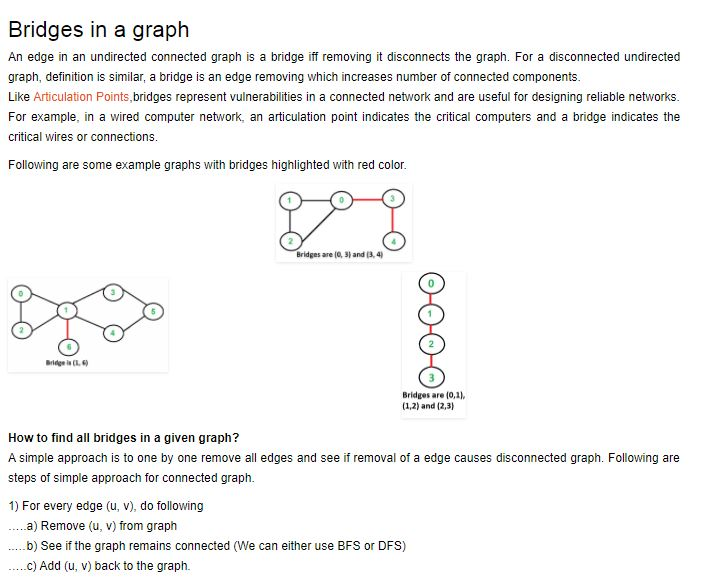// A Java program to find bridges in a given undirected graph
import java.io.*;
import java.util.*;

// This class represents a undirected graph using adjacency list
// representation
class Graph
{
private int V; // No. of vertices

// Array of lists for Adjacency List Representation
int time = 0;
static final int NIL = -1;

// Constructor
Graph(int v)
{
V = v;
for (int i=0; i<v; ++i)
}

// Function to add an edge into the graph
{
}

// A recursive function that finds and prints bridges
// using DFS traversal
// u –> The vertex to be visited next
// visited[] –> keeps tract of visited vertices
// disc[] –> Stores discovery times of visited vertices
// parent[] –> Stores parent vertices in DFS tree
void bridgeUtil(int u, boolean visited[], int disc[],
int low[], int parent[])
{

// Count of children in DFS Tree
int children = 0;

// Mark the current node as visited
visited[u] = true;

// Initialize discovery time and low value
disc[u] = low[u] = ++time;

// Go through all vertices aadjacent to this
while (i.hasNext())
{
int v = i.next(); // v is current adjacent of u

// If v is not visited yet, then make it a child
// of u in DFS tree and recur for it.
// If v is not visited yet, then recur for it
if (!visited[v])
{
parent[v] = u;
bridgeUtil(v, visited, disc, low, parent);

// Check if the subtree rooted with v has a
// connection to one of the ancestors of u
low[u] = Math.min(low[u], low[v]);

// If the lowest vertex reachable from subtree
// under v is below u in DFS tree, then u-v is
// a bridge
if (low[v] > disc[u])
System.out.println(u+” “+v);
}

// Update low value of u for parent function calls.
else if (v != parent[u])
low[u] = Math.min(low[u], disc[v]);
}
}

// DFS based function to find all bridges. It uses recursive
// function bridgeUtil()
void bridge()
{
// Mark all the vertices as not visited
boolean visited[] = new boolean[V];
int disc[] = new int[V];
int low[] = new int[V];
int parent[] = new int[V];

// Initialize parent and visited, and ap(articulation point)
// arrays
for (int i = 0; i < V; i++)
{
parent[i] = NIL;
visited[i] = false;
}

// Call the recursive helper function to find Bridges
// in DFS tree rooted with vertex ‘i’
for (int i = 0; i < V; i++)
if (visited[i] == false)
bridgeUtil(i, visited, disc, low, parent);
}

public static void main(String args[])
{
// Create graphs given in above diagrams
System.out.println(“Bridges in first graph “);
Graph g1 = new Graph(5);
g1.bridge();
System.out.println();

System.out.println(“Bridges in Second graph”);
Graph g2 = new Graph(4);
g2.bridge();
System.out.println();

System.out.println(“Bridges in Third graph “);
Graph g3 = new Graph(7);
g3.bridge();
}
}
output:
Bridges in first graph
3 4
0 3

Bridges in second graph
2 3
1 2
0 1

Bridges in third graph
1 6

3)longestpath()
// method prints longest path of given tree
void Graph::longestPathLength()
{
pair<int, int> t1, t2;

// first bfs to find one end point of
// longest path
t1 = bfs(0);

// second bfs to find actual longest path
t2 = bfs(t1.first);

cout << “Longest path is from ” << t1.first << ” to ”
<< t2.first << ” of length ” << t2.second;
}

output:
Longest path is from 5 to 7 of length 5

#)Breadth-First Graph Traversal Algorithm.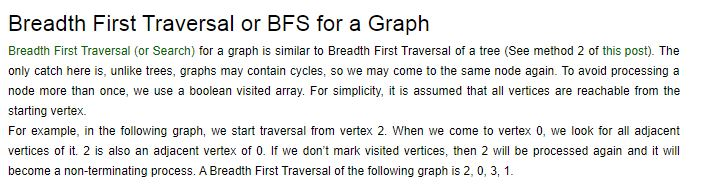// Java program to print BFS traversal from a given source vertex.

// BFS(int s) traverses vertices reachable from s.
import java.io.*;
import java.util.*;

// This class represents a directed graph using adjacency list
// representation
class Graph
{
private int V; // No. of vertices

// Constructor
Graph(int v)
{
V = v;
for (int i=0; i<v; ++i)
}

// Function to add an edge into the graph
{
}

// prints BFS traversal from a given source s
void BFS(int s)
{
// Mark all the vertices as not visited(By default
// set as false)
boolean visited[] = new boolean[V];

// Create a queue for BFS

// Mark the current node as visited and enqueue it
visited[s]=true;

while (queue.size() != 0)
{
// Dequeue a vertex from queue and print it
s = queue.poll();
System.out.print(s+” “);

// Get all adjacent vertices of the dequeued vertex s
// If a adjacent has not been visited, then mark it
// visited and enqueue it
while (i.hasNext())
{
int n = i.next();
if (!visited[n])
{
visited[n] = true;
}
}
}
}

// Driver method to
public static void main(String args[])
{
Graph g = new Graph(4);

System.out.println(“Following is Breadth First Traversal “+
“(starting from vertex 2)”);

g.BFS(2);
}
}
output:
Following is Breadth First Traversal (starting from vertex 2)
2 0 3 1

#)Depth-First Graph Traversal Algorithm.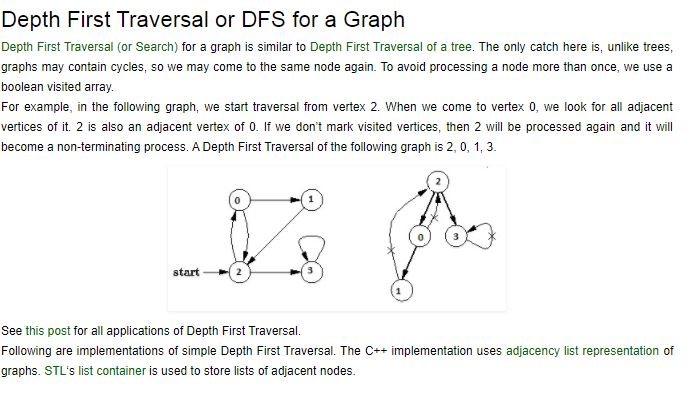// Java program to print DFS traversal from a given given graph
import java.io.*;
import java.util.*;

// This class represents a directed graph using adjacency list
// representation
class Graph
{
private int V; // No. of vertices

// Array of lists for Adjacency List Representation

// Constructor
Graph(int v)
{
V = v;
for (int i=0; i<v; ++i)
}

//Function to add an edge into the graph
{
}

// A function used by DFS
void DFSUtil(int v,boolean visited[])
{
// Mark the current node as visited and print it
visited[v] = true;
System.out.print(v+” “);

// Recur for all the vertices adjacent to this vertex
while (i.hasNext())
{
int n = i.next();
if (!visited[n])
DFSUtil(n, visited);
}
}

// The function to do DFS traversal. It uses recursive DFSUtil()
void DFS(int v)
{
// Mark all the vertices as not visited(set as
// false by default in java)
boolean visited[] = new boolean[V];

// Call the recursive helper function to print DFS traversal
DFSUtil(v, visited);
}

public static void main(String args[])
{
Graph g = new Graph(4);

System.out.println(“Following is Depth First Traversal “+
“(starting from vertex 2)”);

g.DFS(2);
}
}
output:
Following is Depth First Traversal (starting from vertex 2)
2 0 1 3

#)ijkstra’s algorithm for a distance traversal of a Graph from source vertex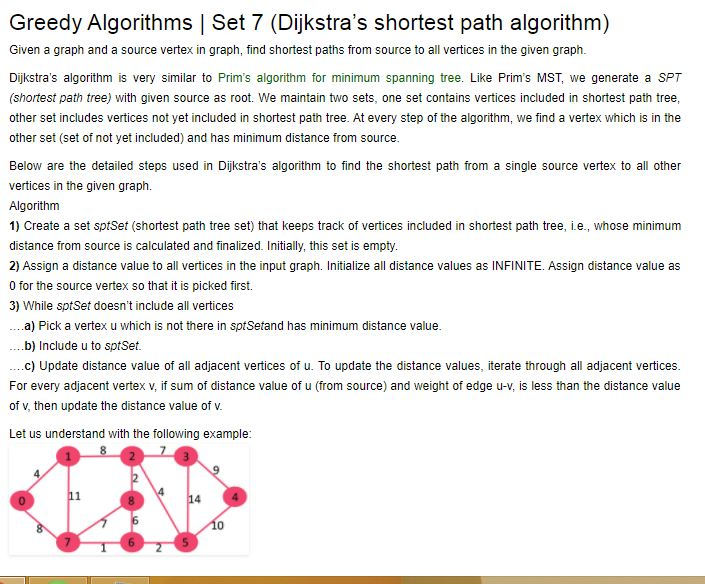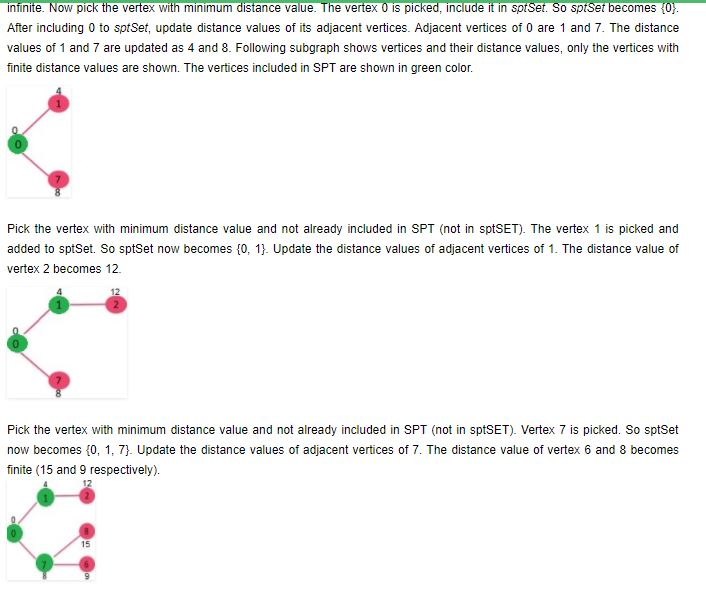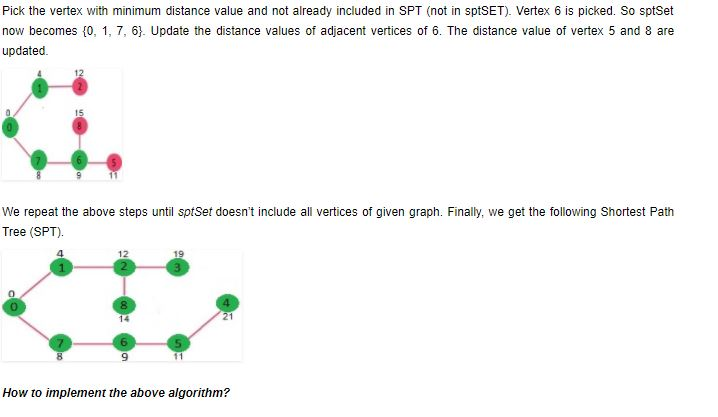// A Java program for Dijkstra’s single source shortest path algorithm.
// The program is for adjacency matrix representation of the graph
import java.util.*;
import java.lang.*;
import java.io.*;

class ShortestPath
{
// A utility function to find the vertex with minimum distance value,
// from the set of vertices not yet included in shortest path tree
static final int V=9;
int minDistance(int dist[], Boolean sptSet[])
{
// Initialize min value
int min = Integer.MAX_VALUE, min_index=-1;

for (int v = 0; v < V; v++)
if (sptSet[v] == false && dist[v] <= min)
{
min = dist[v];
min_index = v;
}

return min_index;
}

// A utility function to print the constructed distance array
void printSolution(int dist[], int n)
{
System.out.println(“Vertex Distance from Source”);
for (int i = 0; i < V; i++)
System.out.println(i+” tt “+dist[i]);
}

// Funtion that implements Dijkstra’s single source shortest path
// algorithm for a graph represented using adjacency matrix
// representation
void dijkstra(int graph[][], int src)
{
int dist[] = new int[V]; // The output array. dist[i] will hold
// the shortest distance from src to i

// sptSet[i] will true if vertex i is included in shortest
// path tree or shortest distance from src to i is finalized
Boolean sptSet[] = new Boolean[V];

// Initialize all distances as INFINITE and stpSet[] as false
for (int i = 0; i < V; i++)
{
dist[i] = Integer.MAX_VALUE;
sptSet[i] = false;
}

// Distance of source vertex from itself is always 0
dist[src] = 0;

// Find shortest path for all vertices
for (int count = 0; count < V-1; count++)
{
// Pick the minimum distance vertex from the set of vertices
// not yet processed. u is always equal to src in first
// iteration.
int u = minDistance(dist, sptSet);

// Mark the picked vertex as processed
sptSet[u] = true;

// Update dist value of the adjacent vertices of the
// picked vertex.
for (int v = 0; v < V; v++)

// Update dist[v] only if is not in sptSet, there is an
// edge from u to v, and total weight of path from src to
// v through u is smaller than current value of dist[v]
if (!sptSet[v] && graph[u][v]!=0 &&
dist[u] != Integer.MAX_VALUE &&
dist[u]+graph[u][v] < dist[v])
dist[v] = dist[u] + graph[u][v];
}

// print the constructed distance array
printSolution(dist, V);
}

// Driver method
public static void main (String[] args)
{
/* Let us create the example graph discussed above */
int graph[][] = new int[][]{{0, 4, 0, 0, 0, 0, 0, 8, 0},
{4, 0, 8, 0, 0, 0, 0, 11, 0},
{0, 8, 0, 7, 0, 4, 0, 0, 2},
{0, 0, 7, 0, 9, 14, 0, 0, 0},
{0, 0, 0, 9, 0, 10, 0, 0, 0},
{0, 0, 4, 14, 10, 0, 2, 0, 0},
{0, 0, 0, 0, 0, 2, 0, 1, 6},
{8, 11, 0, 0, 0, 0, 1, 0, 7},
{0, 0, 2, 0, 0, 0, 6, 7, 0}
};
ShortestPath t = new ShortestPath();
t.dijkstra(graph, 0);
}
}

output:
Vertex Distance from Source
0 0
1 4
2 12
3 19
4 21
5 11
6 9
7 8
8 14

Note: the distance of undirected graph is also covered..